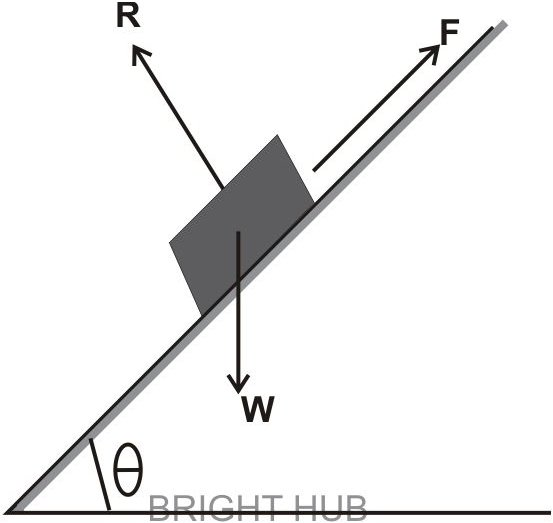# What is Friction? Forces of Friction

Any surface may only appear to be smooth in the relative sense. A wooden table may appear to be very smooth to us, but if look it with an “ant’s” view, you will find the surface filled with numerous dents. Thus no surface can ideally produce perfect smoothness.

The relative degree of roughness associated with all surfaces has the tendency of developing some interlocking property with another surface coming in direct contact with it. So long as the surfaces are stationary, the interlocking does not produce any reaction However when the surfaces move or tend to slide in opposite directions, the interlocking property becomes active and opposes the motion of the surfaces.

This opposing force generated between a moving surface sliding or rubbing against another surface is actually the force of friction, also known as simply friction.

These may be distinguished as Static Friction and Dynamic Friction.

## Types of Frictional Forces

• Static Friction: As discussed above, static friction may exist across two surfaces in contact which are stationery or showing no movements.
• Dynamic Friction: Conversely, dynamic friction may be understood as the friction witnessed across surfaces in motion. Dynamic friction is also known as kinetic friction.
• Limiting Friction: Visualize a body positioned over a surface. A casual push to the body might not produce any movement or displacement on the position of the body, despite the little force that’s being exerted over it. This is probably due to the frictional force which opposes or restricts the body from advancing.

The analysis shows that the frictional force succeeds in countering or balancing the external force and therefore may be assumed to be either equal or more than the external force acting over the body.

It might be further witnessed that even a slight increase in the applied force is unable to move the body, proving that the frictional force has also increased proportionally, succeeding further in maintaining the equilibrium conditions.

The above considerations prove that the force of friction is self adjusting and effectively balances the varying externally applied forces through opposite magnitudes of frictional forces. However, the frictional force fails to sustain the equilibrium when the applied force crosses a certain limit and when the body ultimately begins moving.

This limit which just breaks the maximum frictional force and initiates the slide of the body is called limiting friction.

It may be taken into account that when the applied force over a body is less than the limiting friction, the body may be under the influence of static friction, whose magnitude may be anywhere above zero and the limiting friction.

## Laws of Friction

The great researcher Prof. Coulomb inferred a few laws of static and dynamic friction after doing some thorough assessments, which are as follows:

Laws of Static Friction

• Static friction is a force of friction which always acts in the direction opposite to which the body would have moved in the absence of this force of friction.
• The magnitude of force of static friction is exactly equal to the applied external force which tends the body to move.
• The ratio between the limiting friction force and the normal reactions between the involved surfaces is maintained at a constant level.

Therefore, F/R = Constant

Where F = limiting friction and R = normal reaction.

• The area of constant does not have any influence on force of friction and it is thus independent of it.
• The force of friction is influenced by the degree of roughness of the surfaces.

Laws of Kinetic Friction

• It is a force which acts in the direction opposite to the movement of the body.
• The ratio between kinetic friction force and the reactions normal to the surfaces is held at constant proportions; however it may be a bit less than with static frictions.
• At reasonable speeds, the force of kinetic friction may stay constant, but some what decreases with increase in speeds.

What is Angle of Friction?Referring to the figure, suppose a weight W is resting over an inclined plane. The weight finds itself in equilibrium position under the action of the following forces:

• The weight of the body directed vertically on the plane.
• The force of friction F acting upwards along the plane,
• Normal reaction R acting perpendicular to the plane.

Now suppose, if the angle of inclination Ɵ is gently tilted to increase it, the body at some point may just start moving downward along the plane. This angle of friction which just initiates the sliding action on the body is called the angle of inclination.

Coefficient of Friction

The ratio of normal reaction and the limiting force happening between the surfaces of two bodies is called coefficient of friction, symbolized as µ and presented as:

µ = tan Φ = F/R,

or, F = µR,

where µ = Coefficient of friction,

Φ = Angle of friction,

F = Maximum force of friction,

R = Normal reaction between the two bodies.